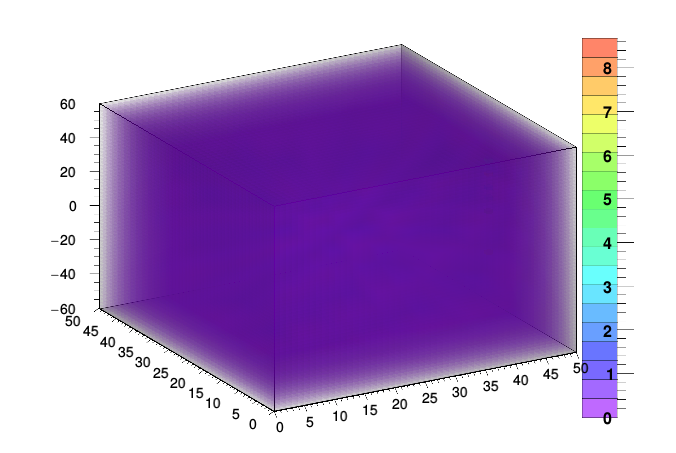# Glcolz in TH3 drawing question

Hello,
Ive been able to fill and draw a “4d scatter plot” using TH3, however it seems to draw empty bins, ie i would like to hide all bins with a zero value so i can actually view the ones of importance, Ive searched around with no luck, I apologize if this is a repeat question.
Im using version 5.34/32, thank you in advance.

heres a snippet:

``````
gROOT->Reset();

TH3F *hist = new TH3F("", "", 50, 0., 50., 50, 0., 50., 60, -60., 60.);
gStyle->SetCanvasPreferGL(true);

for(int i = 0; i < ftime.size(); ++i)
{
lop = ftime.at(i);
time = lop.m;
x = lop.x;
y = lop.y;
z = lop.z;
hist->Fill(x,y,z,time);
}

hist->Draw("glcolz");``````Looking at it

Thanks for the reply, I managed to find what i was looking for here:
[url]TH3 and glcolz option in Oven_Homogeneity.C (posted by tpochep) a function is defined for making empty bins transparent